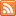Content Tagged “Probability Trees”Conditional Probability and Independence

Published: Sep 19th, 2013

View Lecture Slides with Transcript – Conditional Probability and Independence Part 1 (14:55) Slides 1-11 Part 2 (13:18) Slides 12-18 This document is linked from Conditional Probability and Independence.Conditional Probability and Independence

Published: Jul 28th, 2012

Independent Events Multiplication Rule for Independent Events (Rule Six) Conditional Probability (Rule Seven) Independent Events (Part 2) General Multiplication Rule (Rule Eight) Let’s Summarize CO-6: Apply basic concepts of probability, random […]